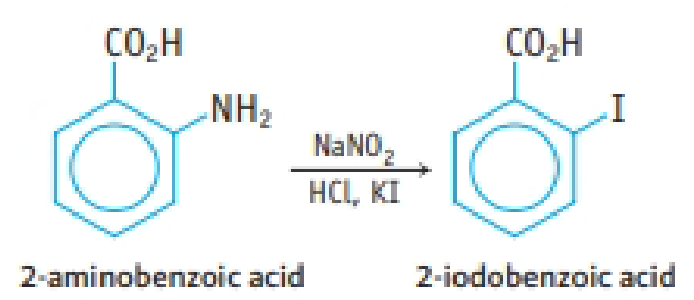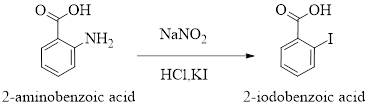Chapter 23, Problem 112IL

Chapter
Section
Textbook Problem

2-Icdobenzoic acid, a tan, crystalline solid, can be prepared from 2-aminobenzoic acid. Other required reagents are NaNO2 and KI (as well as HCI).(a) If you use 4.0 g of 2-aminobenzoic acid, 2.2 g of NaNO2, and 5.3 g of KI, what is the theoretical yield of 2-iodoberaoic acid?(b) Are other isomers of 2-iodobenzoic acid possible?(c) You titrate the product in a mixture of water and ethanol. If you use 15.62 mL of 0.101 M NaOH to titrate 0.399 g of the product, what is its molar mass? Is it in reasonable agreement with the theoretical molar mass?

(a)

Interpretation Introduction

Interpretation: The theoretical yield of 2-iodobenzoicacid has to be determined.

Concept introduction:

Number of moles of a substance,

From its given mass is,

Number of moles=GivenmassMolecularmass

Theoretical yield is the maximum product yield that can be expected based on the masses of the reactants and the reaction stoichiometry.

2-Iodobenzoic acid is a crystaline solid and it can be prepared from 2-aminobenzoic acid  treated with NaNO2, HCl and KI.

The reaction can be represnted as follows,Explanation

From the given data,

2-amino benzoic acid = 4.0 gMolar mass of 2-amino benzoic acid =137.14 g/mol

Let’s calculate the number of moles of 2-amino benzoic acid:

Number of moles = MassMolarmass=4.0 g137.14 g/mol= 0.02916 moles

From the given:

Mass of NaNO2 = 2.2 gMolar mass of NaNO2 = 69 g/mol

Let’s calculate the number of moles of NaNO2:

Number of moles =MassMolarmass=2.2 g69 g/mol= 0.03188 moles

From the given:

Mass of KI = 5

(b)

Interpretation Introduction

Interpretation: To Check the possibilty of isomers for 2-iodobenzoic acid

Concept introduction:

2-Iodobenzoic acid is a crystaline solid and it can be prepared from 2-aminobenzoic acid  treated with NaNO2, HCl and KI.

The reaction can be represnted as follows,Two compounds that have the same molecular formula, but have different structural formulas are called isomers.

(c)

Interpretation Introduction

Interpretation: The molar mass of the product formed in the given reaction has to be determined. And check whether the calculated molar mass is in reasonable agreement with the theoretical molar mass or not.

Concept introduction:

2-Iodobenzoic acid is a crystaline solid and it can be prepared from 2-aminobenzoic acid  treated with NaNO2, HCl and KI.

The reaction can be represnted as follows,Concentration of solutions can be expressed in various terms; molarity is one such concentration expressing term.

Molarity (M) of a solution is the number of gram moles of a solute present in one liter of the solution.

Molarity=MassperlitreMolecular mass

A solution containing one gram mole or 0.1 gram of solute per litre of solution is called molar solution.

Amount of substance (mol) can be determined by using the equation,

Numberofmole=GivenmassofthesubstanceMolarmass

The molar mass of an element or compound is the mass in grams of 1 mole of that substance, and it is expressed in the unit of grams per mol (g/mol).

Theoretical yield is the maximum product yield that can be expected based on the masses of the reactants and the reaction stoichiometry.

Still sussing out bartleby?

Check out a sample textbook solution.

See a sample solution

The Solution to Your Study Problems

Bartleby provides explanations to thousands of textbook problems written by our experts, many with advanced degrees!

Get Started

Find more solutions based on key concepts